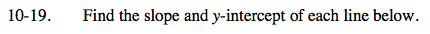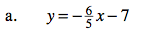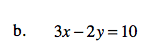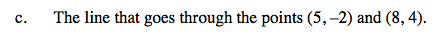### Home > AC > Chapter 10 > Lesson 10.1.2 > Problem10-19

10-19.The equation is already in slope-intercept ( y = mx + b ) form.

m = slope
b = y-intercept

$\text{slope }= -\frac{6}{5}$

y-intercept = (0, −7)Solve the equation for y.
If you are having trouble, refer to part (a).Start by finding the slope.

$\text{Slope }= \frac{4-(-2)}{8-5}=\frac{6}{3}=2$

Substitute a point into the equation
y = mx +b and solve for b, the y-intercept.
4 = 2(8) + b
4 = 16 + b
−12 = b

Slope = 2
y-intercept = (0, −12)